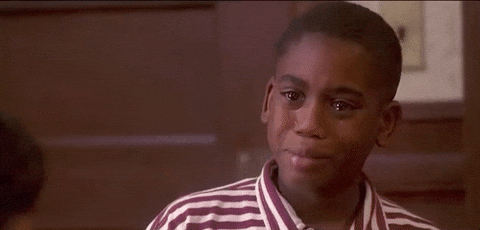# If You Hate Mathematics Please Don’t Take This Quiz#### 1.Correct! Wrong!

Reciprocate the last 2 numbers.

#### 2.Correct! Wrong!

Apple=5, Grapes=4 and Orange=3. 5+5+3-4= 9

#### 3.Correct! Wrong!

The first number on the left and the last number on the right gives you the answer.

#### 4.Correct! Wrong!

Echo logo=10, Vodafone Logo=5 and Kuulpeeps Logo= 3. Therefore, 5+3x8= 80

#### 5.Correct! Wrong!

first digit x first digit and third digit x third digit (4x4 and 3x3). 4x4=16 and 3x3=9. Therefore answer is 169

#### 6.Correct! Wrong!

P=20. Then apple = 40, banana = 20, plum = 5. So the result is 5 + 20 = 25.

#### 7.Correct! Wrong!

First digit on the left and digit on the right

Maths
Well done!You're indeed a maths shark!